×#### Thank you for registering.

One of our academic counsellors will contact you within 1 working day.

Click to Chat

1800-1023-196

+91-120-4616500

CART 0

• 0

MY CART (5)

Use Coupon: CART20 and get 20% off on all online Study Material

ITEM
DETAILS
MRP
DISCOUNT
FINAL PRICE
Total Price: Rs.

There are no items in this cart.
Continue Shopping• Complete JEE Main/Advanced Course and Test Series
• OFFERED PRICE: Rs. 15,900
• View Details

```Chapter 8: Quadratic Equations Exercise – 8.1

Question: 1

(i) x2  - 3x + 2 = 0 , x = 2 , x = - 1

(ii)  x2 + x + 1 = 0, x = 0, x = 1(v) 2x2 - x + 9 = x2 + 4x + 3, x = 2 and x = 3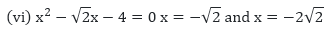(vii) a2x2 - 3abx + 2b2 = 0

Solution:

(i) Here LHS = x2 - 3x + 2 RHS = 0

Now, substitute x = 2 in LHS

We get, (2)2 - 3(2) + 2

= 4 - 6 + 2

= 6 - 6

= 0

RHS Since, LHS =RHS

Therefore, x - 2 is a solution of the given equation.

Similarly, substituting x = - 1 in

LHS

We get, (-1)2 - 3(-1) + 2

= 1 + 3 + 2

= 6

RHS Since, LHS?

RHS = x = - 1 is not the solution of the given equation.

(ii) Here,

LHS = x2  + x + 1 and RHS = 0

Now, substituting x = 0 and x = 1 in

LHS = 02 + 0 + 1

= (1)2 + 1 + 1

= 1 + 2

= 3 LHS

RHS Both x = 0 and x = 1 are not solutions of the given equation.

(iii) Here,

LHS = x2 - 3√3x + 6 = 0 and

RHS = 0 Substituting the value of x = √3 and x = - 2√3 in= 3 – 9 + 6

= 9 - 9

= 0= 12 + 18 + 6

= 36

RHS x = √3 is a solution of the above mentioned equation. Whereas, x = - 2√3 is not a solution of the above mentioned equation.
(iv) Here,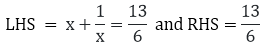Substituting where x = 5/6 and x = 4/3 in the LHS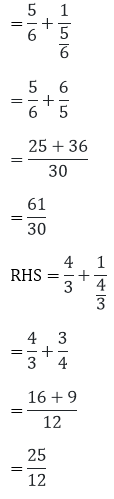RHS where x = 5/6 and x = 4/3 are not the solutions of the given equation.

(v) 2x2 - x + 9 = x2 + 4x + 3, x = 2

= 2x2 - x + 9 - x2 + 4x + 3 = x2 - 5x + 6 = 0

Here, LHS = x2 - 5x + 6 and RHS = 0

Substituting x = 2 and x = 3

= x2 - 5x + 6

= (2)2 - 5(2) + 6

= 10 - 10

= 0

= RHS = x2 - 5x + 6

= (3)2 - 5(3) + 6

= 9 - 15 + 6

= 15 – 15

= 0

= RHS x = 2 and x = 3 both are the solutions of the given quadratic equation.

(vi) Here,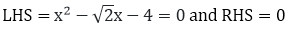Substituting the value x = - √2 and x = - 2√2 in= 2 – 2 – 4

= – 4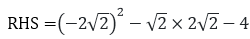= 8 – 4 – 4

= 8 – 8

= 0

= RHS x = - 2√2 is the solution of the above mentioned quadratic equation.

(vii) Here,

LHS = a2x2 - 3abx + 2b2 and RHS = 0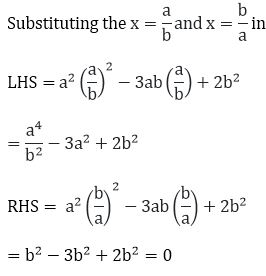RHS = x = b/a

is the solution of the above mentioned quadratic equation.

Question: 2

(i) Given that 2/3 is a root of the given equation. The equation is 7x2 + kx - 3 = 0

(ii) Given that x=a is a root of the given equation x2 - x(a + b) + k = 0

(iii)  Given that x = √2 is a root of the given equation kx2 + √2x - 4

(iv) Given that x = - a is the root of the given equation x2 + 3ax + k = 0

Solution:

(i) Given that 2/3 is a root of the given equation. The equation is 7x2 + kx - 3 = 0

According to the question 2/3 satisfies the equation.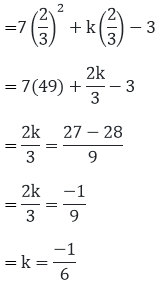(ii) Given that x = a is a root of the given equation x2 - x(a + b) + k = 0 = x = a satisfies the equation =  a2- a(a + b) + k = 0

= a2 - a2- ab + k

K = ab

(iii) Given that x = √2 is a root of the given equation kx2 + √2x - 4

x = √2 satisfies the given quadratic equation.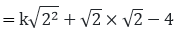= 2k + 2 – 4 = 0

= 2k – 2 = 0

k = 1

(iv) Given that x= -a is the root of the given equation x2 + 3ax + k = 0

Therefore, = (- a)2 + 3a(- a) + k = 0

= a2 + 3a2 + k = 0

= k = 4a2= - a satisfies the equation

Question: 3

Given to check whether 3 is a root of the equation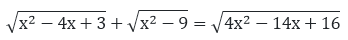Solution: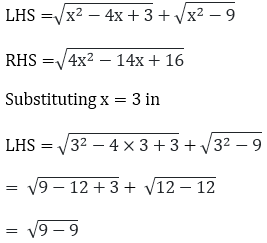= 0

Similarly putting x = 3 in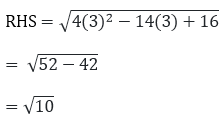LHS ≠ RHS

x = 3 is not the solution the given quadratic equation.
```### Course Features

• 728 Video Lectures
• Revision Notes
• Previous Year Papers
• Mind Map
• Study Planner
• NCERT Solutions
• Discussion Forum
• Test paper with Video Solution